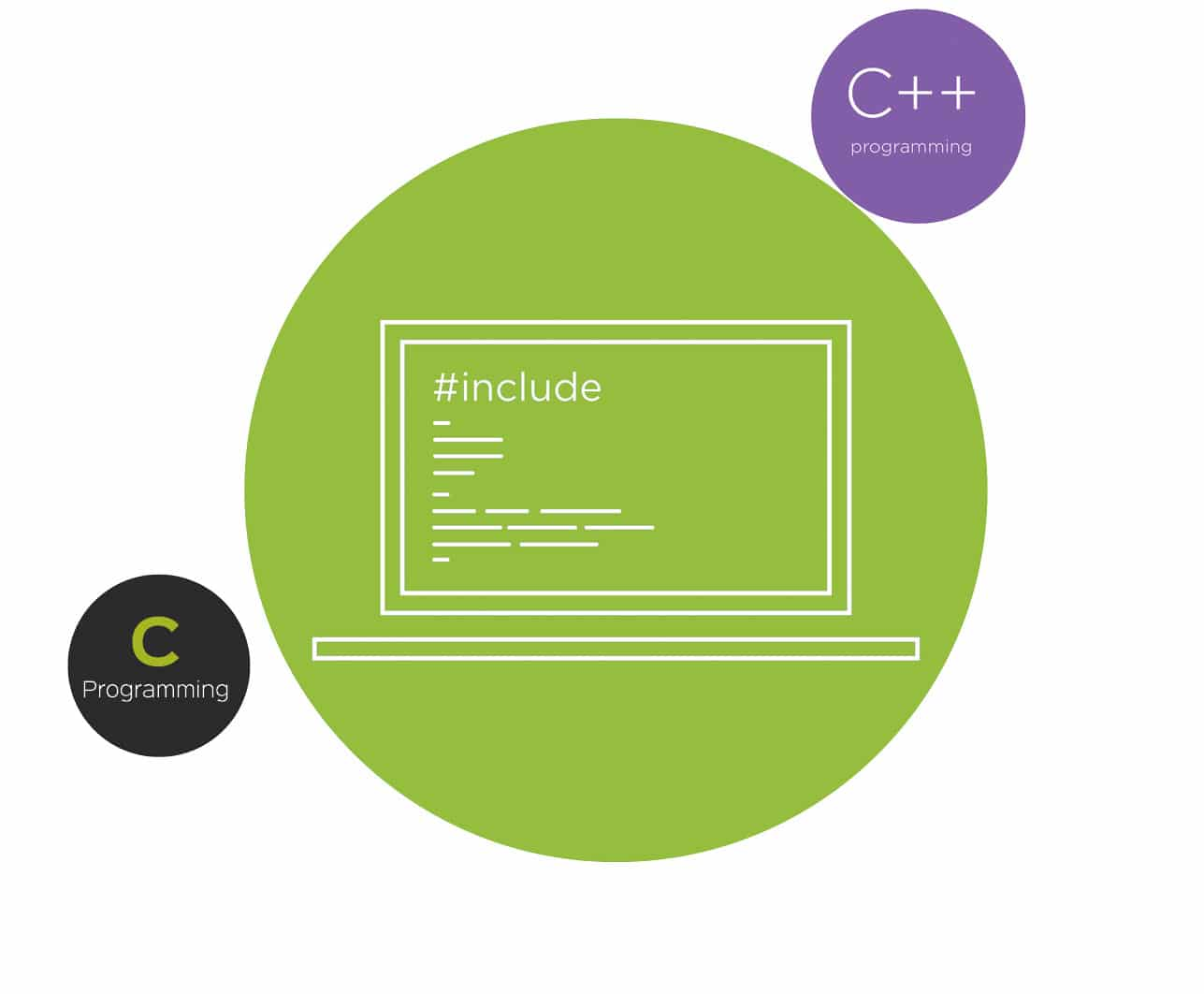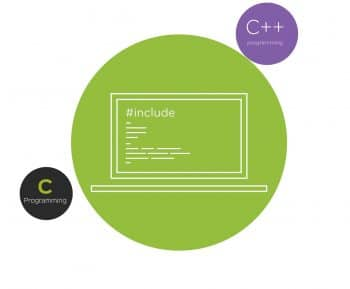Minor differences between C and C++

0
6842This article discusses the subtle and minute differences between C and C++.

An article titled ‘Major Differences Between C and C++’ would have been quite ridiculous because everybody knows that C and C++ are two independent programming languages, both backed by communities that are quite fanatical in their support for their respective preference. There will probably be a small group of novice programmers who still post questions in StackOverflow with the tag ‘C/ C++’ and will get panned universally by professional programmers belonging to both the camps. In this article, I plan to point out a few subtle differences between C and C++, altogether avoiding the major differences like C being an imperative programming language whereas C++ is an object-oriented programming language. This article tries to point out that even though text books often define C++ as a superset of C, even that part of C++ that is a subset of C is slightly different from traditional C.

I will try to prove the existence of such minute differences between C and C++ that often escape the notice of even seasoned programmers with a small example. This is a question I have asked recently in a programming competition: “Write an error-free C program that will give an error when compiled as a C++ program.” Since the classical definition of C++ says that it is a superset of C, every C program should also compile as a C++ program without any errors. This might be the case theoretically though not so practically. The following program Example 1 fails when compiled with the g++ compiler as a C++ program, but when compiled as a C program with the gcc compiler, it compiles successfully.

```#include<stdio.h>
int main()
{
int class;
}```

Example 1

What is the reason for the error when compiled as a C++ program? That’s very simple—the keyword class in C++ can be used as a variable name in C. But to crown it all, the first prize went to a programming whizz-kid who knew that anonymous structures are okay in C but will lead to an error in C++. The program Example 2 with an anonymous structure will compile as a C program with a warning, whereas in C++ the program will result in an error. All the programs in this article are compiled as C programs with gcc and as C++ programs with g++.

```#include<stdio.h>

struct
{
int a;
};

int main()
{
}```

Example 2

There are many such examples where C and C++ show their differences in the least expected places. For example, the program Example 3 will display different outputs on the screen when compiled as C and C++ programs.

```#include<stdio.h>
int main()
{
int i,j;
i=sizeof(‘a’);
i=--i/3*32+65;
for(j=i;j<i+26;j++)
{
printf(“%c “,j);
}
printf(“\n”);
}```

Example 3

The program when compiled as a C program will output the lower case letters, and the same program will output upper case letters when compiled as a C++ program. This is due to the fact that the size of a character literal is 4 bytes in C and 1 byte in C++, because C treats a character literal as integer type and C++ treats a character literal as character type. Figure 1 shows the output of the program execution as C and as C++.

In C, by default, the return type of a function is an integer. But this default to the int rule is not valid in C++. You have to mention the return type as integer explicitly. Due to this, the program Example 4 will show an error when compiled as a C++ program.

```#include<stdio.h>

fun()
{

}

int main()
{
fun();
}```

Example 4

In C, an empty function prototype tells the compiler that the function can have any number of arguments. But in C++, it means the function cannot take any arguments. For example, the function declaration int fun( ); in C can receive zero or more arguments, whereas in C++, this function has zero arguments. The program Example 5 shows an error when compiled as a C++ program.

```#include<stdio.h>
int fun();
int main()
{
fun(123);
}
int fun(int n)
{
printf(“\n Number is %d\n”,n);
}```

Example 5

The unary increment and decrement operators in C++ return an lvalue. But in C, they return an rvalue. So (++i)++; is valid in C++ but it will lead to an error in C. The program Example 6 will show the error message ‘lvalue required’ in C whereas in C++, the program will successfully compile and show the output as ‘i = 3’.

```#include<stdio.h>

int main()
{
int i=1;
(++i)++;
printf("\ni = %d\n",i);
}```

Example 6

In C, we cannot get the address of a register variable. But in C++, it is possible to get the address of a register variable. The program Example 7 is not an error-free C program but when compiled as C++, the program runs without any errors.

```#include<stdio.h>

int main()
{
register int x=123;
printf(“\n Address of x = %u\n”,&x);
}```

Example 7

In C and C++, static variable initialisation is different. In C, static variables can only be initialised with constant values whereas in C++, this is not necessary. The program Example 8 will not compile as a C program but it is valid when compiled with g++ as a C++ program.

```#include<stdio.h>

int main()
{
int x=123;
static int y=x;
printf(“\ny = %d\n”,y);
}```

Example 8

The way case labels are treated in C and C++ is different. C++ allows constant integer variables as case labels, whereas C will give you an error. The program Example 9 will give an error as a C program but as a C++ program, it compiles without any errors.

```#include<stdio.h>

int main()
{
int i=1;
const int j=1;
switch(i)
{
case j:
printf(“\nWorks for C++\n”);
break;
case 2:
printf(“\nError for C\n”);
break;
default:
printf(“\nCheck by compiling\n”);
}
}```

Example 9

In C, uninitialised constant variables are just fine but these will lead to an error in the case of C++. For example, a program with the line of code const int a; will compile fine as a C program, whereas it will show a compile time error when compiled as C++ and display the error message uninitialized const. Another difference arises when the keyword typedef is used in C and C++. C++ treats the names of struct, union, enum, etc, as implicit typedef names. This is not the case with C, so program Example 10 is a valid C program, whereas if compiled as a C++ program, you will get the error message using typedef-name ‘type’ after ‘struct’.

```#include<stdio.h>

typedef int type;
struct type
{
type a;
};
struct type sv;

int main()
{
}```

Example 10

In C, a pointer of type void is automatically cast into the target pointer type when assigned to a pointer of any other type. So, there is no need for explicit type-casting in C. Whereas in C++, explicit type-casting is required when a void pointer is assigned to a pointer of another type. So, the line int *ptr = malloc(sizeof(int)); is fine in C, whereas in C++, you will get the compile time error message: invalid conversion from ‘void*’ to ‘int*’. You can download this and all the other programs discussed in this article from opensourceforu.com/article_source_code/june17cPlus.zip. To save some space, I haven’t used any code other than the feature being discussed in many of the programs. But you can add any valid C or C++ code in all these programs.

K & R style function definitions are still valid in C. But this will lead to an error in case of C++. So, the program Example 11 is a valid C program but it is not a valid C++ program.

```#include<stdio.h>
void fun(a, b)
int a;
int b;
{
printf(“\na = %d \nb = %d\n”,a,b);
}
int main()
{
fun(11,22);
}```

Example 11

In C++, goto or switch cannot cross an initialisation. The program Example 12 works fine as a C program but fails to compile as a C++ program. In this program, goto is crossing an initialisation, which is an error in C++. The error message crosses initialization of ‘int i’ is displayed when the program is treated as C++.

```#include<stdio.h>
int main()
{
goto jump_over_initialization;
int i=0;
printf("\nI will not be printed\n");
jump_over_initialization:
}```

Example 12

Similarly, refer the program switch.c, in which the error is due to the fact that switch is crossing an initialisation. In C, an enum variable can take any value, whereas in C++, only the values specified in the declaration can be assigned to an enum variable. In C, a character array overflow is tolerated with a warning, whereas C++ is stricter about this matter and will directly lead to a compile time error. But, of course, the option -Werror of gcc, if enabled, will result in a compile time error for the program array.c in C also. The result of a Boolean expression is different in C and C++. The program Example 13 will print 4 as the output when treated as a C program and 1 as the output when treated as a C++ program.

```#include<stdio.h>
int main()
{
printf(“\n%d\n”, sizeof(1==1));
}```

Example 13

The size of a structure with no members is different in C and C++. The program Example 14 gives 0 as the output when treated as a C program and 1 as the output when treated as a C++ program.

```#include<stdio.h>
struct empty
{
};
int main()
{
printf(“\n%d\n”, sizeof(struct empty));
}```

Example 14

I hope these minor differences between C and C++ pointed out in this article will help students and computer professionals in technical interviews. But do remember that this article does not cover all such differences and I will be much obliged if somebody could point out any other such differences that are not covered in this article. Before concluding, I would like to acknowledge my brilliant student, Julin Shaji, for identifying many of these subtle differences between C and C++.